# High School Math : Using Limits with Continuity

## Example Questions

### Example Question #1 : Using Limits With Continuity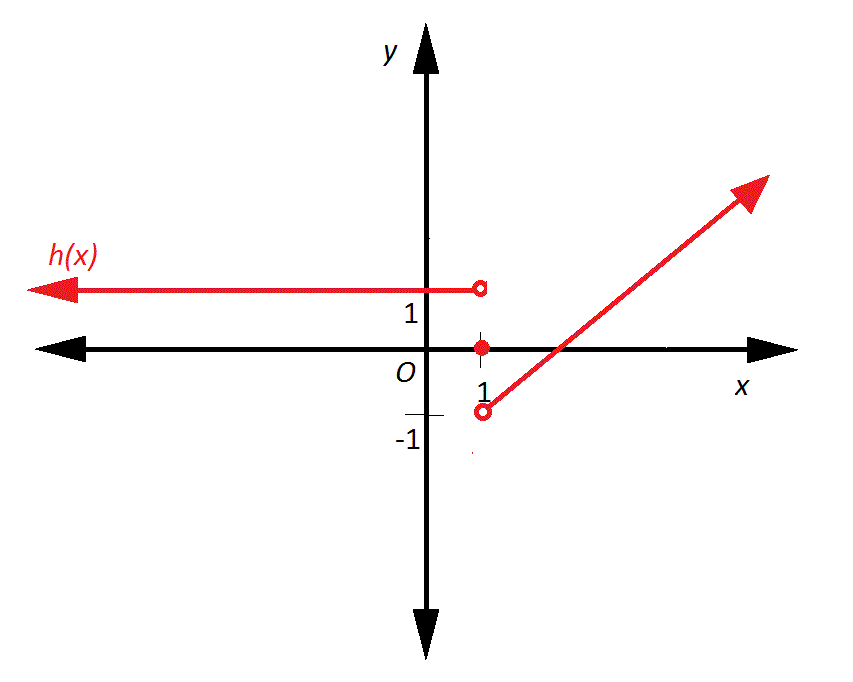The above graph depicts a function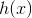. Does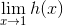exist, and why or why not?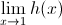does not exist because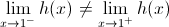.exists because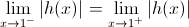does not exist because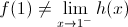exists because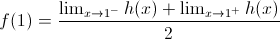does not exist because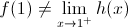does not exist because.

Explanation:exists if and only if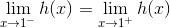. As can be seen from the diagram,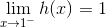, but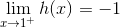. Since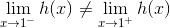,does not exist.

### Example Question #2 : Using Limits With Continuity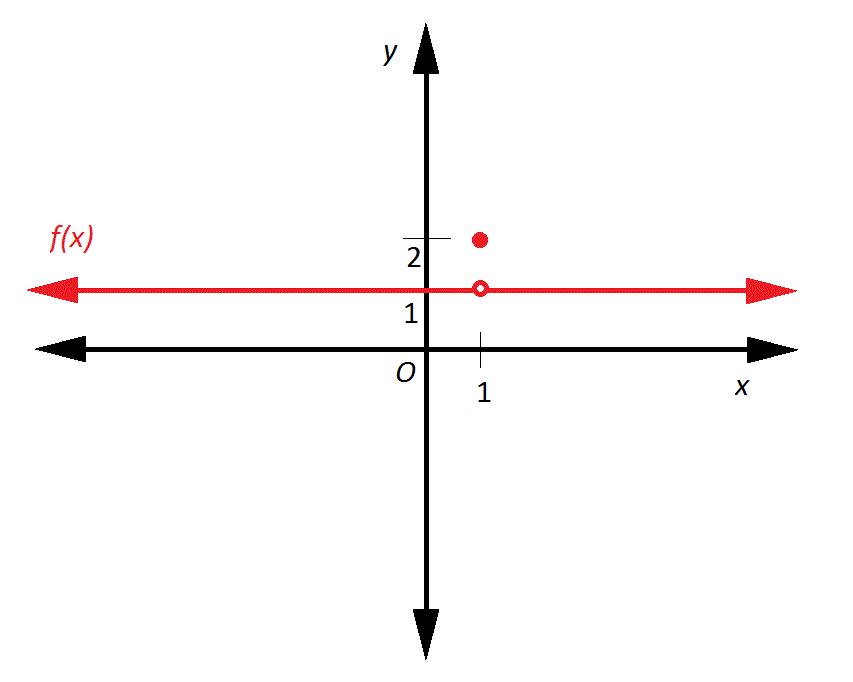The above graph depicts a function. Does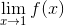exist, and why or why not?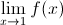does not exist because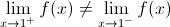does not exist because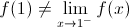does not exist because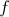is not continuaous at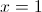.exists because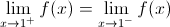does not exist because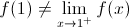exists becauseExplanation:exists if and only if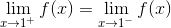;

the actual value ofis irrelevant, as is whetheris continuous there.

As can be seen,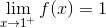and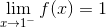;

therefore,,

andexists.

### Example Question #3 : Using Limits With Continuity

A function is defined by the following piecewise equation: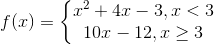At, the function is:

discontinuous

continuous

continuous

Explanation:

The first step to determine continuity at a point is to determine if the function is defined at that point. When we substitute in 3 for, we get 18 as our-value.is thus defined for this function.

The next step is determine if the limit of the function is defined at that point. This means that the left-hand limit must be equal to the right-hand limit at. Substitution reveals the following: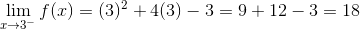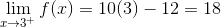Both sides of the function, therefore, approach a-value of 18.

Finally, we must ensure that the curve is smooth by checking the limit of the derivative of both sides.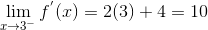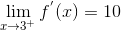Since the function passes all three tests, it is continuous.

### Example Question #4 : Using Limits With Continuity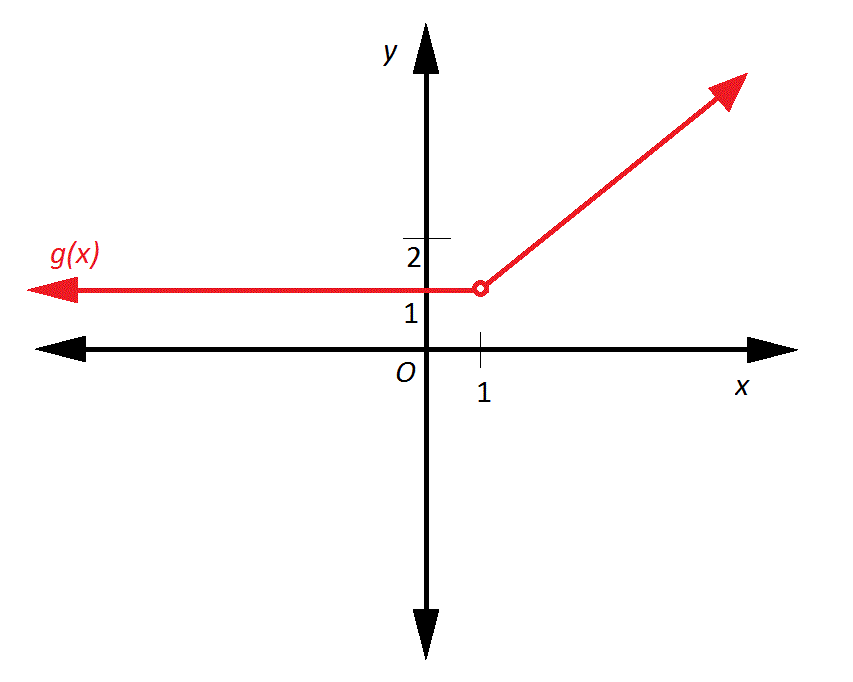The graph depicts a function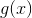. Does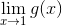exist?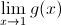does not exist because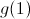is undefined.exists because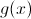is constant on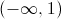.exists because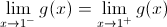.does not exist because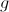is not continuous at.does not exist because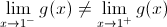.exists because.exists if and only if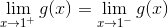; the actual value of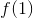is irrelevant.
As can be seen,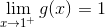and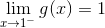; therefore,, andexists.